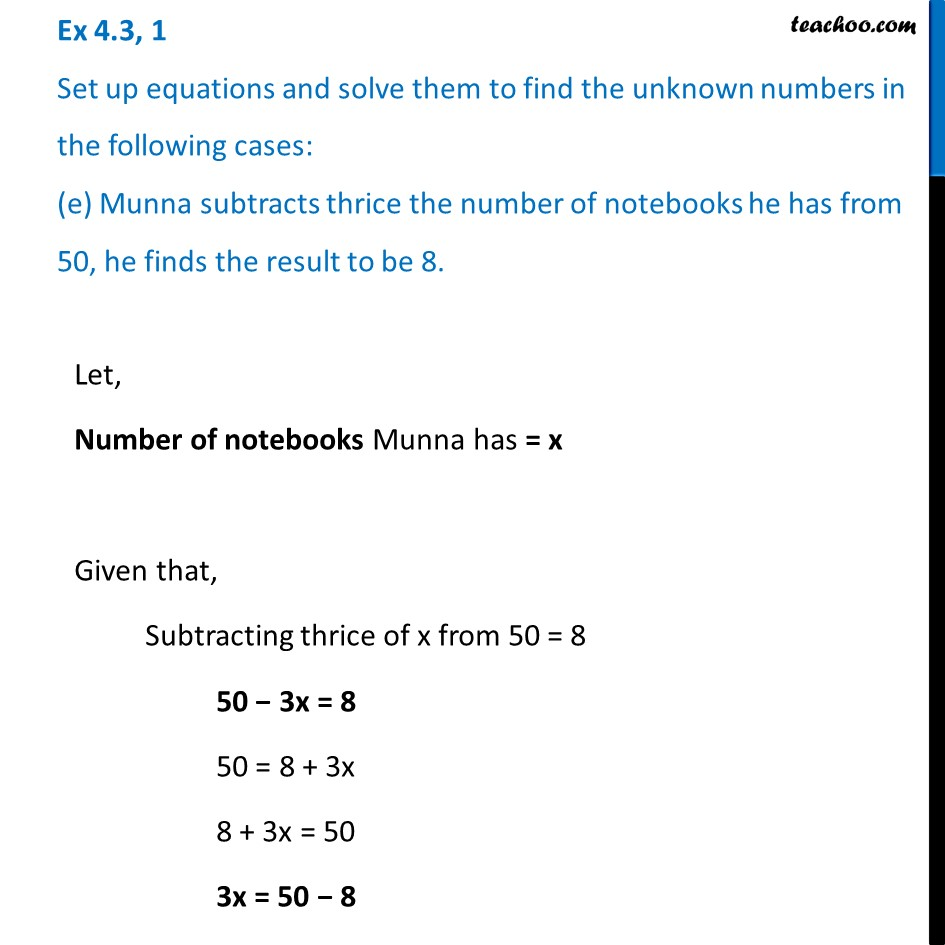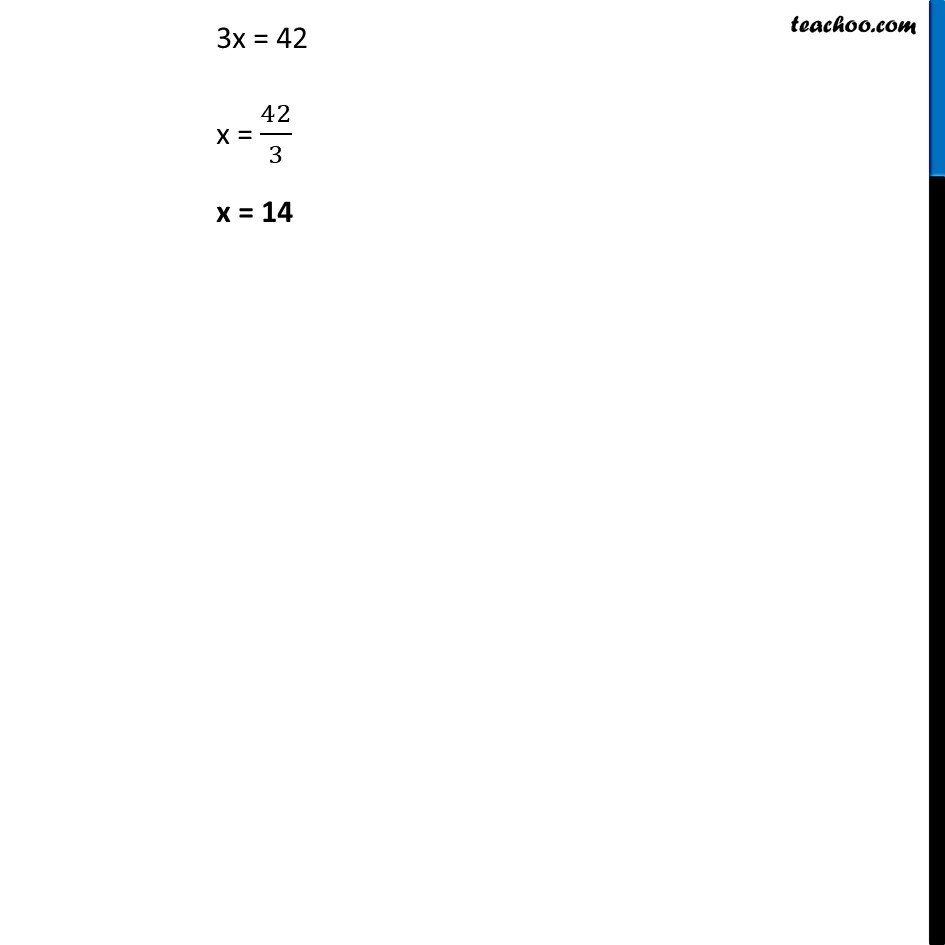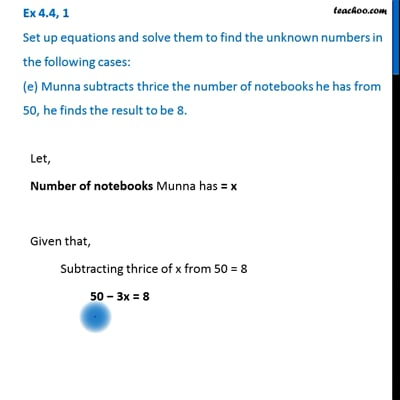Ex 4.3

Chapter 4 Class 7 Simple Equations
Serial order wiseThis video is only available for Teachoo black users

Learn in your speed, with individual attention - Teachoo Maths 1-on-1 Class

### Transcript

Ex 4.3, 1 Set up equations and solve them to find the unknown numbers in the following cases: (e) Munna subtracts thrice the number of notebooks he has from 50, he finds the result to be 8.Let, Number of notebooks Munna has = x Given that, Subtracting thrice of x from 50 = 8 50 − 3x = 8 50 = 8 + 3x 8 + 3x = 50 3x = 50 − 8 3x = 42 x = 42/3 x = 14